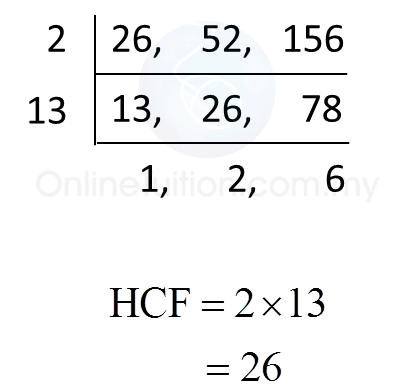# 2.2.4 Number Patterns and Sequences, PT3 Practice

 [adinserter block="3"]       
Question 16:
 State the first two common multiples of 3, 5 and 6.
 
 Solution:
 
Multiples of 3 = 3, 6, 9, 12, 15, 18, 21, 24, 27, 30, 33, 36, 39, 42, 45, 48, 51, 54, 57, 60, …
 Multiples of 5 = 5, 10, 15, 20, 25, 30, 35, 40, 45, 50, 55, 60, …
 Multiples of 6 = 6, 12, 18, 24, 30, 36, 42, 48, 54, 60, …
 
 The first two common multiples of 3, 5 and 6 are 30 and 60.
 
 
 
 [adinserter block="3"]       
Question 17:
 Find the lowest common multiple (LCM) of 4, 8 and 24.
 
 Solution:[adinserter block="3"]       
Question 18:
 Find the lowest common multiple (LCM) of 8, 12 and 15.
 

 Solution:[adinserter block="3"]   
Question 19:
 Find the highest common factor (HCF) of 26, 52 and 156.
 

 Solution:[adinserter block="3"]   
Question 20:
 Given that (m + 5) is the highest common factor of 81 and 108, find the value of m.
 

 Solution: